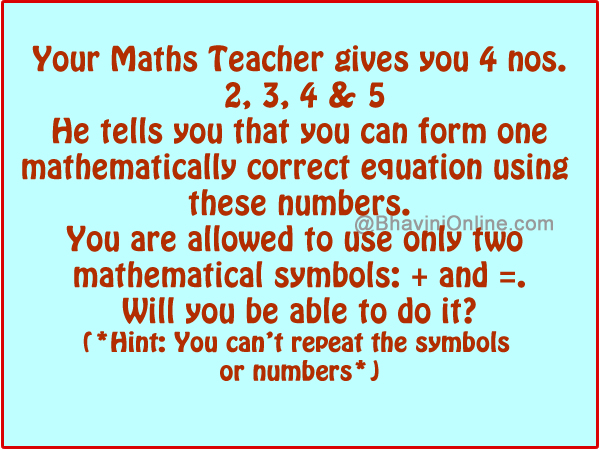# Fun Math Riddles: Find Correct Equation with the Numbers 2, 3, 4 & 5

Solve this fun math riddle and leave your answers in the comment section.

Your Mathematics Teacher gives you four numbers;

2, 3, 4 & 5

He tells you that you can form one mathematically correct equation using these numbers.

You are allowed to use only two mathematical symbols: + and =.

Will you be able to do it?

Hint: You can’t repeat the symbols or numbers.So were you able to solve the riddle? Leave your answers in the comment section below.

## 3 Replies to “Fun Math Riddles: Find Correct Equation with the Numbers 2, 3, 4 & 5”

1. Atharva says:

4+5=3²

2. A says:

Or alternatively, 3+4=2+5?

1. Jatin says:

Riddles are interesting.